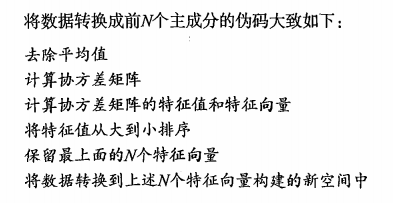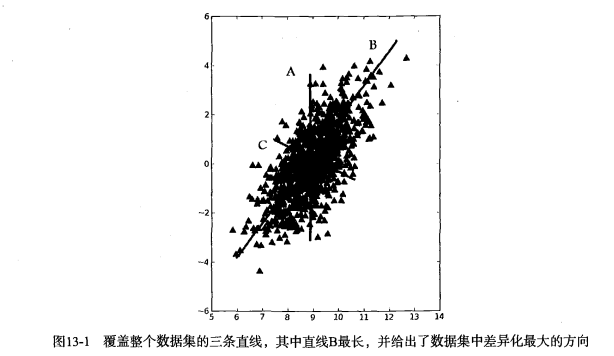# 降维技术

## PCA(Principal Component Analysis)主成分分析# 基本统计学概念

## 协方差是什么

cov(X,Y)=ni=1(XiX¯¯¯)(YiY¯¯¯)n1

## 协方差矩阵是什么

C=cov(x,x)cov(y,x)cov(z,x)cov(x,y)cov(y,y)cov(z,y)cov(y,z)cov(y,z)cov(z,z)$C=\begin{equation} \left( \begin{array}{ccc} cov(x,x) & cov(x,y) & cov(y,z)\\ cov(y,x) & cov(y,y) & cov(y,z)\\ cov(z,x) & cov(z,y) & cov(z,z)\\ \end{array} \right) \end{equation}$

## PCA与协方差矩阵

PCA的理念就是将高维空间的数据映射到低维空间，降低维度之间的相关性，并且使自身维度的方差尽可能的大。那么哪种数据方式可以同时表达维度之间的相关性以及，维度本身的方差呢？就是上面提到的协方差矩阵。协方差矩阵对角线上是维度的方差，其他元素是两两维度之间的协方差(即相关性)。
PCA的目的之一：降低维度之间的相关性，也就说减小协方差矩阵非对角线上的值。如何减小呢？可以使协方差矩阵变成对角矩阵。对角化后的矩阵，其对角线上是协方差矩阵的特征值。这里又提到了特征值，看看它到底有啥潜在的含义。

## 特征值与特征向量

Ax矩阵相乘的含义就是，矩阵A对向量x进行一系列的变换(旋转或者拉伸)，其效果等于一个常数c乘以向量x。# code

from numpy import *

fr=open(fileName)
datArr=[map(float,line) for line in stringArr]
return mat(datArr)

def pca(dataMat,topNfeat=9999999):
#原始数据归一化
meanVals=mean(dataMat,axis=0)
meanRemoved=dataMat-meanVals
#求协方差矩阵，python真方便，一大坨计算，一个函数搞定
covMat=cov(meanRemoved,rowvar=0)
#求特征值，特征向量
eigVals,eigVects=linalg.eig(mat(covMat))
#特征值排序
eigValInd=argsort(eigVals)
eigValInd=eigValInd[:-(topNfeat+1):-1]
redEigVects=eigVects[:,eigValInd]
#原始数据映射到新的维度空间
lowDataMat=meanRemoved*redEigVects
reconMat=(lowDataMat*redEigVects.T)+meanVals
return lowDataMat,reconMat

# end

©️2019 CSDN 皮肤主题: 编程工作室 设计师: CSDN官方博客